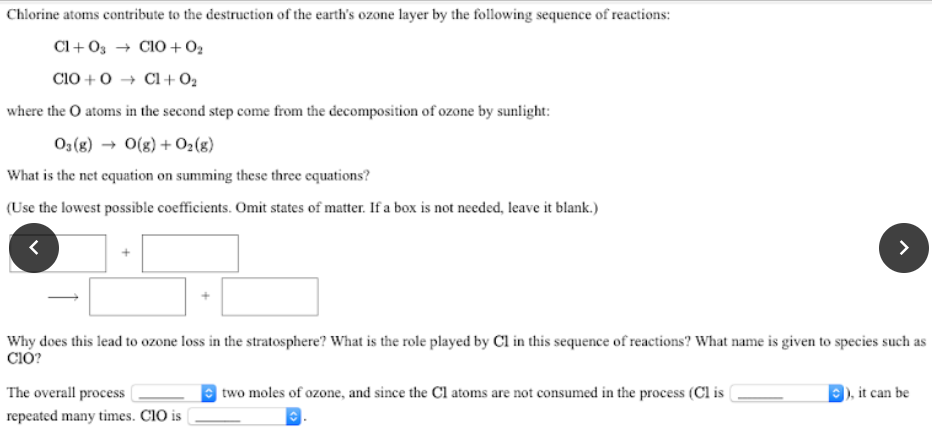# Chlorine atoms contribute to the destruction of the earth's ozone layer by the following sequence of reactions: Cl + O3 -> ClO + O2; ClO + O -> Cl + O2 where the O atoms in the second step come from the decomposition of ozone by sunlight: O3(g) -> O(g) + O2(g) What is the net equation on summing these three equations? Why does this lead to ozone loss in the stratosphere? What is the role played by Cl in this sequence of reactions? What name is given to species such as ClO?